# 折射## 射入密度较大的介质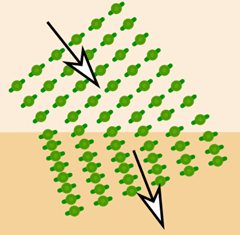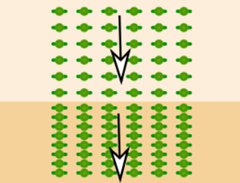## 射入密度较小的介质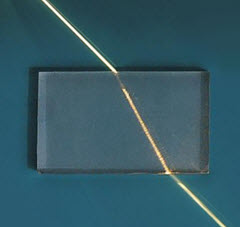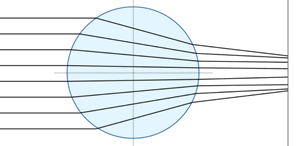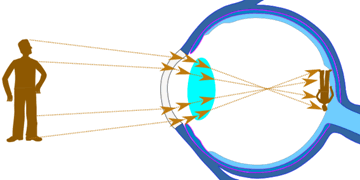## 折射率

n = c v

• n 是折射率
• c 是真空里的速度
• v 是介质里的速度

### 例子：水的折射率是 1.333

• 真空里的速度：300,000千米每秒
• 水里的速度：300,000千米每秒 / 1.333 = 225,000千米每秒

n

228 1.31
225 1.333

## 临界角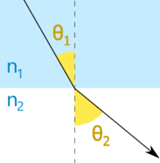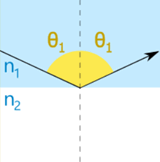折射 临界角 全内 反射

### 例子：水到空气## 斯涅尔定律

n1 sin(θ1) = n2 sin(θ2)

 n1sin(θ1) = n2sin(θ2) n1sin(θcrit) = n2 θ1 = θ1 因为 sin(90°)=1 当 θ1 > θcrit折射 临界角 全内 反射

### 例子：空气与水的临界角是多大？

 开始：: n1 sin(θ1) = n2 sin(θ2) sin(90°) = 1，所以： n1 sin(θcrit) = n2 重排： sin(θcrit) = n1 n2 重排： θcrit = sin-1( n1 n2 ) 代入数值： θcrit = sin-1( 1.003 1.333 ) 计算： θcrit = 48.8°

n1 n2 = sin(θ2) sin(θ1)

（小心：n 的值是 "1 除以 2"，但 sin() 的值是 "2 除以 1".)